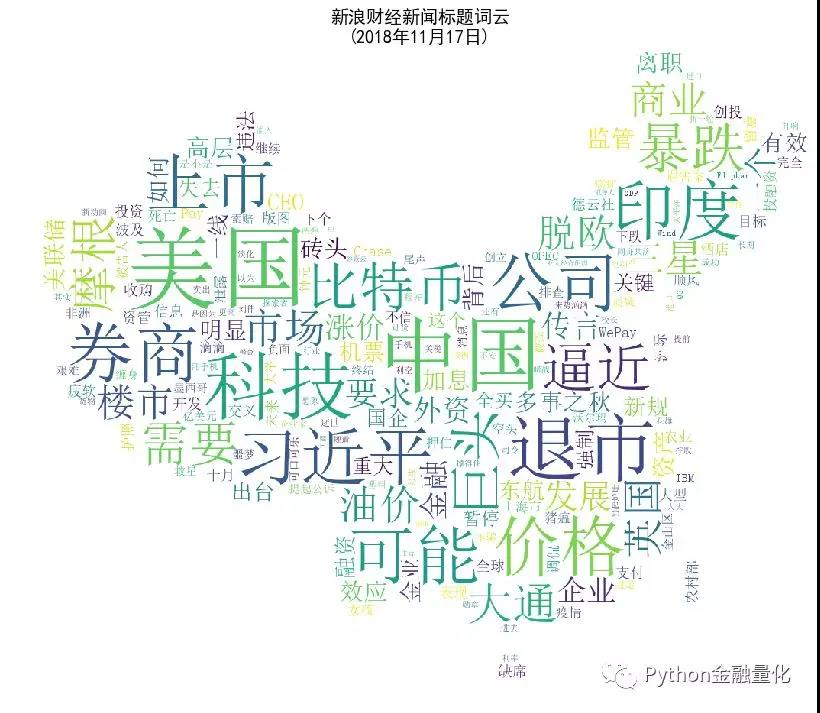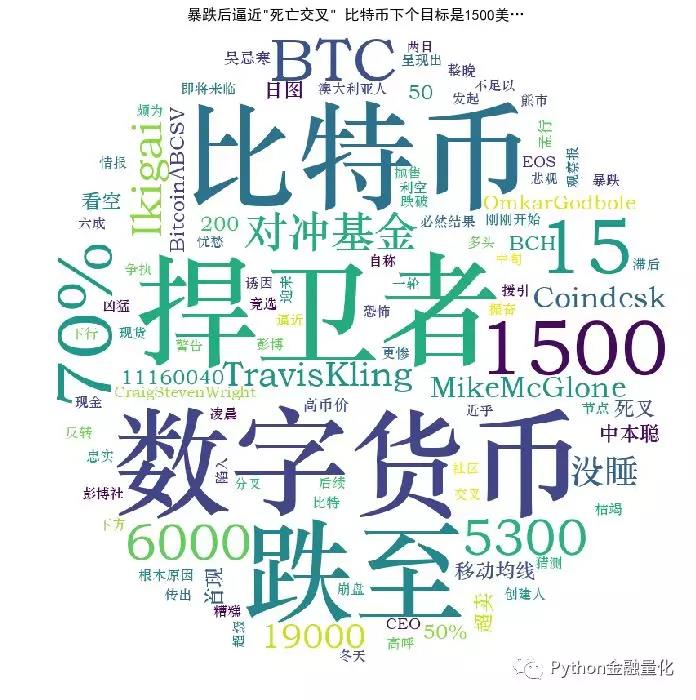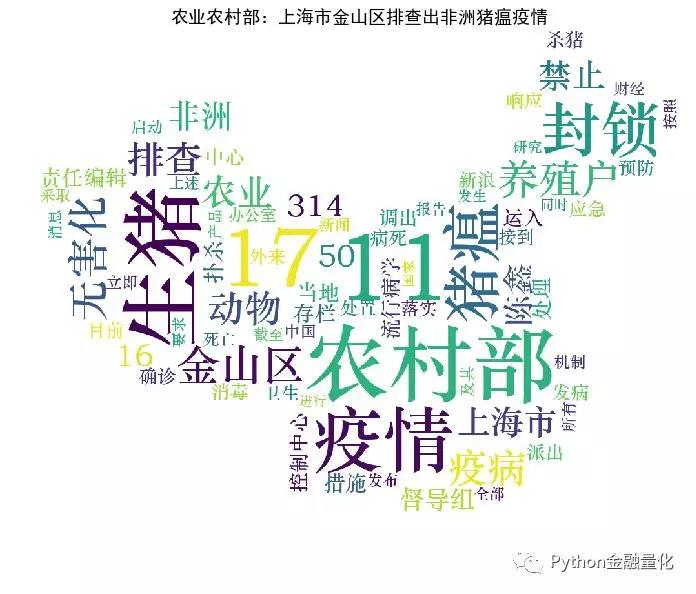# 【Python金融量化】财经新闻文本分析

“80%的商业信息来自非结构化数据，主要是文本数据”（Seth
Grimes），这一说法可能夸大了文本数据在商业数据中的占比，但是文本数据所蕴含的信息价值是毋庸置疑的。在信息爆炸的社会，文本数据量如此庞大，我们能做什么呢？事实上，能做的有很多，主要取决于你的目标是什么。

tushare是目前比较好用的数据开源包，可以免费获取股票、期货、宏观、基本面等数据，之后的金融量化分析实践基本上都会使用该包来获取数据，具体使用方法，可以到tushare的官网查看。

``#注意：黑色方框背景里的代码可以左右滑动查看 #引入需要用到的包 #金融量化分析常用到的有：pandas（数据结构）、 #numpy（数组）、matplotlib（可视化）、scipy（统计） import tushare as ts import pandas as pd import matplotlib.pyplot as plt %matplotlib inline import jieba import jieba.analyse from wordcloud import WordCloud, STOPWORDS, ImageColorGenerator #正常显示画图时出现的中文和负号 from pylab import mpl mpl.rcParams['font.sans-serif']=['SimHei'] mpl.rcParams['axes.unicode_minus']=False df=ts.get_latest_news(show_content=True) #获取当前即时财经新闻（如本文是2018年11月17日） #默认是80条，可以通过参数“top= ”来设置 #保存数据到本地 #df.to_csv("D:/CuteHand/news.csv",encoding='gbk') #加encoding='gbk'才不会中文乱码，如果存在“非法字符”,可能也会报错 #数据清洗，保留需要的字段 df=df[['time','title','content']] #查看前三条数据 df.head(3) ````#提取新闻标题内容并转化为列表（list） #注意原来是pandas的数据格式 mylist = list(df.title.values) #对标题内容进行分词（即切割为一个个关键词） word_list = [" ".join(jieba.cut(sentence)) for sentence in mylist] new_text = ' '.join(word_list) #图片可以根据需要更改，这里使用了中国地图.jpg #读取图片 img = plt.imread("中国地图.jpg") #设置词云格式 wc = WordCloud(background_color="white", mask=img,#设置背景图片 max_font_size=120, #字体最大值 random_state=42, #颜色随机性 font_path="c:\windows\fonts\simsun.ttc") #font_path显示中文字体，这里使用黑体 #生成词云 wc.generate(new_text) image_colors = ImageColorGenerator(img) #设置图片大小 plt.figure(figsize=(14,12)) plt.imshow(wc) plt.title('新浪财经新闻标题词云\n(2018年11月17日)',fontsize=18) plt.axis("off") plt.show() #将图片保存到本地 #wc.to_file("财经新闻标题词云.jpg") ````#以第一条新闻内容为例（比特币暴跌新闻） #数据清洗 #将titles列专门提取出来，并转化为列表形式 d=list(df.content) content=''.join(d) #设置分词黑名单，手动暴力解决 #以后探索使用机器学习和深度学习进行分词 blacklist = ['责任编辑', '\n','\t', '也', '上', '后', '前', '为什么', '再', ',','认为','12','美元', '以及', '因为', '从而', '但', '像','更', '用', '“', '这', '有', '在', '什么', '都','是否','一个' ,'是不是','”', '还', '使', '，', '把', '向','中', '新', '对', ' ', ' ', u')', '、', '。', ';', '之后','表示','%', '：', '?', '...', '的','和', '了', '将', '到', ' ',u'可能','2014','怎么', '从', '年', '今天', '要', '并', 'n', '《', '为', '月', '号', '日', '大','如果','哪些', '北京时间', '怎样', '还是', '应该','这个', '这么','没有','本周','哪个', '可以','有没有'] #将某些固定词汇加入分词 stopwords=['比特币','利空','对冲基金', '分析师','移动均线','数字货币','中本聪'] for word in stopwords: jieba.add_word(word) #设置blacklist黑名单过滤无关词语 d = {} #将词语转入字典 for word in jieba.cut(content): if word in blacklist: continue if len(word)<2: #去除单个字的词语 continue d[word] = d.get(word, 0) + 1 #使用jieba.analyse d=''.join(d) tags=jieba.analyse.extract_tags(d,topK=100, withWeight=True) tf=dict((a,a) for a in tags) backgroud_Image = plt.imread('比特币.jpg') wc = WordCloud( background_color='white', # 设置背景颜色 mask=backgroud_Image, # 设置背景图片 font_path='C:\Windows\Fonts\STZHONGS.TTF', # 若是有中文的话，这句代码必须添加 max_words=2000, # 设置最大现实的字数 stopwords=STOPWORDS,# 设置停用词 max_font_size=150,# 设置字体最大值 random_state=30) wc.generate_from_frequencies(tf) plt.figure(figsize=(12,12),facecolor='w',edgecolor='k') plt.imshow(wc) # 是否显示x轴、y轴下标 plt.title(df.title,fontsize=15) plt.axis('off') plt.show() ````#将上述词云作图包装成函数 def plot_news_cloud(df,n): txt= [line.strip() for line in df.content[n]] text=''.join(txt) tags=jieba.analyse.extract_tags(text,topK=100, withWeight=True) tf=dict((a,a) for a in tags) backgroud_Image = plt.imread('中国地图.jpg') #可以自己找适合的图片做背景，最后是背景白色 wc = WordCloud( background_color='white', # 设置背景颜色 mask=backgroud_Image, # 设置背景图片 font_path='C:\Windows\Fonts\STZHONGS.TTF', # 若是有中文的话，这句代码必须添加 max_words=2000, # 设置最大现实的字数 stopwords=STOPWORDS,# 设置停用词 max_font_size=150,# 设置字体最大值 random_state=30) wc.generate_from_frequencies(tf) plt.figure(figsize=(12,10),facecolor='w',edgecolor='k') plt.imshow(wc) plt.title(df.title[n],fontsize=18) plt.axis('off') plt.show() #画出第6条新闻的词云图 plot_news_cloud(df,5) ````def word_processing(text): #数据清洗，限于篇幅，代码省略 def sentiment_score_list(dataset): #数据处理和情绪判断主函数， #限于篇幅，代码省略 def sentiment_score(senti_score_list): #情绪得分汇总 #将上述新闻标题去掉空格，写入列表里（list） y=[] t1=list(df.title) for i in range(len(t1)): x=t1[i].split() x=','.join(x) if i<len(t1)-1: x=x+'。' y.append(x) #显示前三条新闻标题 y[:3] ``

[‘暴跌后逼近”死亡交叉”,比特币下个目标是1500美…。’, ‘全球资管巨头：未来5年投资，你需要留意这5件事。’,
‘洲际酒店被指泄露花总护照信息并调侃,波及德云社。’]

``#将上述标题内容合成一起，以句号结尾 wlist=[line.strip() for line in y] wlist=''.join(wlist) #wlist senti_score_list=sentiment_score_list(wlist) text=sentiment_score(senti_score_list) print(text) p=0 n=0 for i in range(len(text)): if text[i]>0: p+=1 else: n+=1 print("正面新闻数目：{0},负面新闻数目：{1}".format(p,n)) ``

[-33, 11, -1, 12, -9, 0, -6, 0, -8, -5, -8, 8.0,14, 13, 0, -2.0, 7,
3, 9, -8, 3, -3, 4, -4, 8, 22,19, 11, -11, -16, 6,13, 11, 9, 0, -9,
12, 2, 4,12,-10, 6, 2, -4, -6, -13, 7,-9, 0, -28, 11, -10, -4, -13,
0, -3, -1, 0, 0, 7,9,15,12,7, 7, 0, 1, 0, 0,0, 12.0, -9, 15, -11, 1,
-9, 1, -6, 9, 0] 正面新闻数目：38,负面新闻数目：42